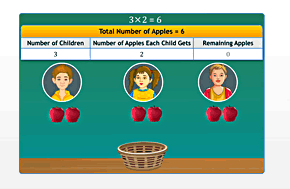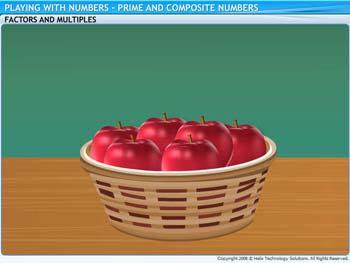## Thursday, 3 April 2014

### Prime and Composite Numbers

Prime and Composite Numbers# Prime and Composite Numbers

An exact divisor of a number is called its factor.
To view the complete lesson click the video below
An exact divisor of a number is called its factor.
Ex: 1, 2, 3 and 6 are factors of number 6.The number 1 is a factor of every number.  Every number is a factor of itself.  The factors of a number are either less than or equal to the number itself.  All numbers have a finite number of factors.  The product of two numbers is called a multiple of each of the two numbers being multiplied.  A number is a multiple of all its factors.  Every number is a multiple of 1 and of itself.  There are infinite multiples of a number. If the sum of the factors of a number is two times the number, then the number is called a perfect number.  Numbers that have only two factors in the form of 1 and the number itself are called prime numbers.

Numbers that have more than two factors are called composite numbers.  The number 1 is neither a prime number nor a composite number.  All numbers with 0, 2, 4, 6 or 8 in the unit’s or one’s place are multiples of 2, and are called even numbers.  All numbers with 1, 3, 5, 7 or 9 in the unit’s or one’s place are called odd numbers
The number 2 is the smallest prime number, and also the only prime number that is even.  All prime numbers, except 2, are odd numbers.   The sum of any two prime numbers, except with 2, is an even number.